Courses

# Mole & Redox Reaction 18-12-2018

## 30 Questions MCQ Test AAKSC Chemistry Mock test Series | Mole & Redox Reaction 18-12-2018

Description
This mock test of Mole & Redox Reaction 18-12-2018 for Class 12 helps you for every Class 12 entrance exam. This contains 30 Multiple Choice Questions for Class 12 Mole & Redox Reaction 18-12-2018 (mcq) to study with solutions a complete question bank. The solved questions answers in this Mole & Redox Reaction 18-12-2018 quiz give you a good mix of easy questions and tough questions. Class 12 students definitely take this Mole & Redox Reaction 18-12-2018 exercise for a better result in the exam. You can find other Mole & Redox Reaction 18-12-2018 extra questions, long questions & short questions for Class 12 on EduRev as well by searching above.
QUESTION: 1

### What is the mass of Fe304 if it reacts completely with 25 ml of 0.3m K2Cr2O7?

Solution: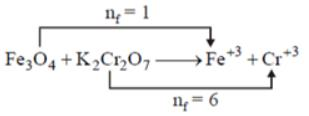neqFe3O4 = neq K2Cr2O7
wM × nf = 0.3 * 25 * 6
w = (0.3×25×6×232)/1
w = 10.44 g.

QUESTION: 2

### One mole of a mixture of CO and CO2 requires exactly 20 g of NaOH in solution for complete conversion of all the CO2 into Na2CO3 .How much NaOH would it require for conversion into Na2CO3, if the mixture (one mole) is completely oxidised to CO2?

Solution:

2 NAOH + CO₂ — > Na₂CO₃ + H₂O

Mole ratio :

1 : 2

We need 2 moles of NaOH to convert one mole of CO₂ to Na₂CO₃.

Moles of NaOH :

20 / 40 = 0.5 moles

Moles of CO₂ :

0.5/ 2 = 0.25

Moles of CO are:

1 - 0.25 = 0.75 moles.

Oxidation of CO :

CO + 1/2 O₂ —> CO₂

Mole ratio is 1 : 1

Moles of CO₂ formed are 0.75 moles.

Thus is half the moles of NaOH required.

NaOH required is thus :

2 × 0.75 =1.5 moles

1 mole — > 40 g

1.5 moles —>?

1.5 × 40 = 60g

60 g is required

QUESTION: 3

### 1.60 g of a metal were dissolved in HNO3 to prepare its nitate. The nitrate on strong heating gives 2 g of its oxide. The equivalent weight of metal is:

Solution:

Eq. of metal = Eq. of oxide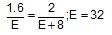QUESTION: 4

The maximum amount of BaSO4 precipitated on mixing 20 mL of 0.5 M BaCI2 with 20 mL of 1 M H2SO

Solution: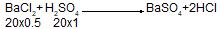mm taken    =10    20        0    0
mm formed    0    10        10    20
Milli mole of BaSO4=10
or
Mole of BaSO4 = 10-2

QUESTION: 5

What is the [OH-) in the final solution prepared by mixing 20.0 mL of 0.050 M HCI with 30.0 mL of 0.10 M Ba(OH)2?

Solution: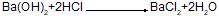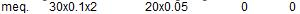=6        =1
5        0        1    1
∴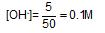QUESTION: 6

Oxidation number of carbon in C3O2, Mg2C3 are respectively:

Solution:

Carbon has negative oxidation no. in Mg3C2 and positive oxidation number in C3O2; O is more electronegative than C. Mg is more electropositive than C.

QUESTION: 7

Which is not a redox reaction?

Solution:

No change in oxidation number of any species.

QUESTION: 8

Which is not correct in case of Mohr’s salt?

Solution:

Ox. no. of Fe in Mohr’s salt,
[FeSO4.(NH4)2SO4.6H2O] is +2

QUESTION: 9

In the reaction;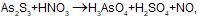the element oxidised is/are

Solution: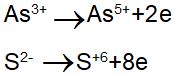QUESTION: 10

HCO-3 contains carbon in the oxidation state:

Solution:

1+ a +3 x (-2) = -1
∴ a = +4

QUESTION: 11

Oxidation number of Fe in Fe0.94O is:

Solution:

0.94 x a +1 x (-2) = 0
∴  a = 200/94

QUESTION: 12

The brown ring complex [Fe(H2O)5NO+]SO4 has oxidation number of Fe:

Solution:

No in iron complex has +1 ox. no. in MnSO4

QUESTION: 13

The pair of compounds which cannot exist in solution is:

Solution:

NaHCO3 being an acid salt will react with NaOH as, one mole of substance.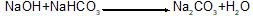QUESTION: 14

0.5 mole of H2SO4 is mixed with 0.2 mole of Ca(OH)2 The maximum number of mole of CaSO4 formed is:

Solution:

Eq. of H2SO4 = 0.5x2=1.0;
Eq. of Ca(OH)2 = 0.2x2 = 0.4;
Equal Eq. reacts and thus, Eq. of CaSO4formed = 0.4
∴ Mole of CaSO4 formed = 0.4/2 = 0.2

QUESTION: 15

Volume of 0.1 M NaOH needed  for the neutralisation of 20 mL of 0.05 M oxalic acid is:

Solution:

Meq. of NaOH = Meq. oxalic acid;
0.1x1xV = 20x0.05x2;

∴ V = 20mL

QUESTION: 16

How many garm of KMnO4 should be taken to make up 250 mL of a solution of such strength that 1 mL is equivalent to 5.0 mg of Fe in FeSO4?

Solution:

Meq. of KMnO4 in 1 mL = Meq. of Fe =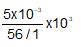∴ Meq. of KMnO4 in 250 mL =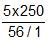Thus,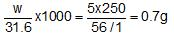QUESTION: 17

25 mL of 0.50 M H2O2 solution is added to 50 mL of 0.20 M KMnO4 in acidic solution. Which of the following statements is true?

Solution:

Meq. of H2O2 = 25x0.5x2 = 25;
Meq. of KMnO4 = 50x0.2x5 = 50;
25 Meq. or 5 milli mole of KMnO4 are left

QUESTION: 18

What volume of 0.40 M Na2S2O3 would be required to react with the I2 liberated by adding excess of KI to 50 mL of 0.20 M CuSO4 solution?

Solution:

Meq. of Na2S2O3 = Meq .of CuSO4
V x0.4x1=50x0.2x1
V = 25 mL

QUESTION: 19

The number of mole of potassium salt, i.e, KHC2O4.H2C2O4.2H2O oxidised by one mole of permanganate ion is:

Solution: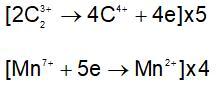QUESTION: 20

5 g of a sample of bleaching  powder is treated with excess acetic acid and KI solution. The liberated I2 required 50 mL of N/10 hypo. The percentage of available chlorine in the sample is:

Solution:

Meq. of bleaching powder = Meq. of CI2 = Meq. of hypo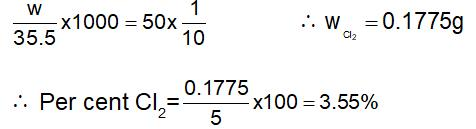QUESTION: 21

4.4 g of CO2  and  2.24 litre of H2 at STP are mixed in a container. The total number of molecules present in the container will be:

Solution: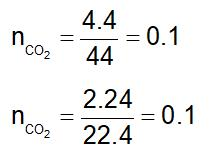QUESTION: 22

20 g of an acid furnishes 0.5 moles of H3O+ ions in its aqueous solution. The value of 1 g eq. of the acid  will be.

Solution:

0.5 mole of H2O+=20 g; Also H3O+  is monovalent, thus
Mol.wt.=Eq.wt.
∴  1 mole of H3O+ = 40 g = GEW of acid

QUESTION: 23

The chloride of a metal contains 71 % chlorine by weight and the vapour density of it is 50. The atomic weight of the metal will be:

Solution:

Mol.wt. of metal chloride = 50x2=100
Let metal chloride be MCIn then
Eq. of metal = Eq. of chloride, or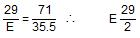Now    a+35.5n =100
or    n.E+35.5n=100;
n = 2
Therefor,    a=2xE = 2x(29/2) = 29

QUESTION: 24

The simplest formula of a compound containing 50% of element X (at. wt. 10) and 50% of element  Y (at. wt. 20) is:

Solution:

g atom of X = 50/10 = 5;
g atom of Y = 50/20 = 2.5;
Ratio of g atom of X and Y = 2:1.

QUESTION: 25

One g  of a mixture of Na2CO3 and NaHCO3 consumes y equivalent of HCI for complete neutralisation. One g of the mixture is strongly heated, then cooled and the residue treated with HCI how many equivalent of HCI would be required for com-plete neutralisation?

Solution: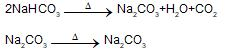QUESTION: 26

The pair of species having same percentage of carbon is:

Solution:

Both have same empirical formula CH2O

QUESTION: 27

0.5 g of fuming H2SO4 (oleum) is diluted with water. This solution is completely neutralised by 26.7 mL of 0.4 N NaOH. The percentage of free SO3 in the sample is:

Solution:

Meq. of H2SO4+Meq. of SO3=Meq. of NaOH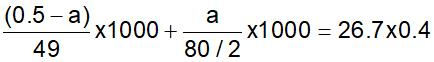∴  a = 0.103
∴  % of SO3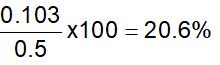QUESTION: 28

Equivalent weight of bivalent metal is 32.7. Molecular weight of its chloride is:

Solution:

mol.wt. of MCI2=2x32.7+71=136.4

QUESTION: 29

If 20% nitrogen is present in a compound, it’s minimum molecular weight will be.

Solution:

20 g N, then mol.wt. =100
14g N, then mol.wt.=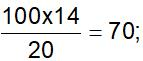QUESTION: 30

0.52 g of a dibasic acid required 100 mL of 0.1 N NaOH for complete neutralisation. The equivalent weight of acid is:

Solution:

Meq. of acid = Meq. of NaOH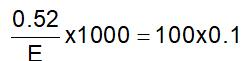∴  E = 52